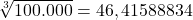Question

If you layered 100000 cubes to make one big cube what would the dimensions of the big cube

1.thuthuy

The dimensions of the cube would be:

• Base: 46,42 units approx.
• Height: 46,42 units approx.
• Width: 46,42 units approx.

Step-by-step explanation:

For this exercise, I suppose the measure of each cube is 1 unit, because there no says what is (you can replace it for ft, in, or another unit you need). How the big cube is formed by the 100.000 cubes smaller, and how a cube has its three measures equal: base, height, and width, you only need to obtain the cubic root of the total cubes (you use cubic root because you’re gonna calculate a cube, for example, if you’d wanna calculate a square, you must obtain the square root of the total cubes):

•How each cube measure is 1 unit, when you calculate the volume of the cube with the value obtained, you must obtain the total of the cubes:

• Volume of the cube= base * height * width
• Volume of the cube= 46,41588834 * 46,41588834 * 46,41588834
• Volume of the cube= 100.000

In this form, you know the answer 46,41588834 is the right answer. I use the number with just two decimals (46,42 approximately) but you can use the complete number if you need.